﻿ “一带一路”货物贸易网络结构动态变化及其影响机制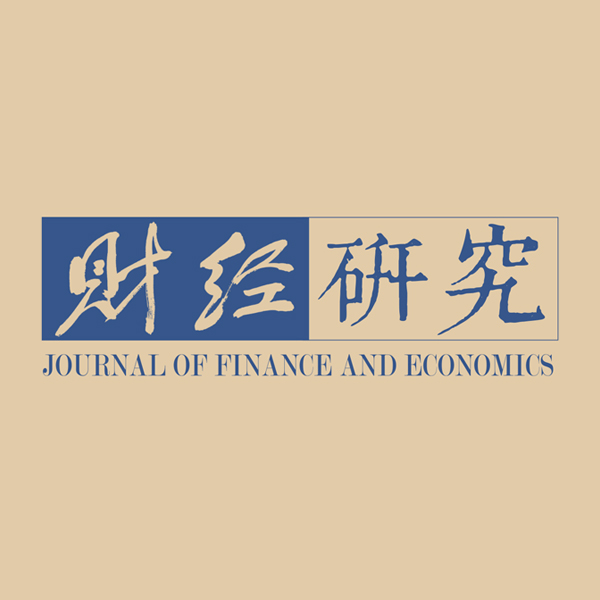《财经研究》
2020第46卷第7期
“一带一路”货物贸易网络结构动态变化及其影响机制

Research on the Dynamic Change of Goods Trade Network Structure and Its Impact Mechanism of Countries along the Belt and Road
Tang Xiaobin, Cui Maosheng
School of Statistics，University of International Business and Economics，Beijing 100029，China
Key words: countries along the Belt and Road    the structure of goods trade network    dynamic change and its impact mechanism    TERGM and STERGM

（一）“一带一路”沿线国家货物贸易网络静态分析方法构建原理

${V}_{i}=\left[{v}_{i}\right]$
i=1，2，3，…，n）表示出口国，
${V}_{j}=\left[{v}_{j}\right]$
j=1，2，3，…，n）表示进口国；设邻接矩阵
$A=\left[{a}_{ij}\right]$
i=1，2，3，…，nj=1，2，3，…，n）表示ij两国之间的贸易关系。对贸易关系阈值的设置参考李敬等人（2017）的做法，若i国对j国存在1亿美元以上的出口贸易额，则
${a}_{ij}$
=1；否则
${a}_{ij}=0$
。用
$G=({V}_{i},{V}_{j},A)$

$\sum\nolimits_{ij}{a}_{ij}$

 $D=\sum\nolimits_{ij}{a}_{ij}/N\left(N-1\right)$ (1)

${a}_{ij}$

 ${OD}_{i}=\sum\nolimits_{i}{a}_{ij}$ (2)
 ${ID}_{i}=\sum\nolimits_{i}{a}_{ji}$ (3)

 $Reciprocity=2\sum\nolimits_{ij}{a}_{ij}{a}_{ji}/\sum\nolimits_{ij}{a}_{ij}$ (4)

 ${CC} = 3{\tau _\Delta }\left( G \right)/{\tau _3}\left( G \right)$ (5)

${\tau }_{\Delta }\left(G\right)$
G中的三角形个数，
${\tau }_{3}（G）$

$M=(V,E)$
（其中VE分别为网络中节点和边的集合），令二元随机变量
${Y}_{ij}={Y}_{ji}$

$e\in E$
，则
${ Y}=$
[
${Y}_{ij}]$
M的邻接矩阵，假设
${ y}=\left[{y}_{ij}\right]$
M的一个特定情况，可以以指数分布族形式定义
${ Y}$

 $Pr \left( {{{Y}} = {{y}}{\rm{|}}{{\theta }}} \right) = \left( {\frac{1}{c}} \right){\rm{exp}}\left\{ {\mathop \sum \nolimits_H {\theta _H}{g_H}\left( {{y}} \right)} \right\}$ (6)

$c=c({ \theta })$

${g}_{H}({ y})$

${\theta }_{H}$

${ \theta }$
${\theta }_{H}$

${\theta }_{0}$
，采用MCMC算法生成大量的图，然后计算生成图分布的各类统计量的期望值是否与实际观察到的网络统计指标值相同，如果存在偏差，就对
${\theta }_{0}$

（二）“一带一路”沿线国家货物贸易网络动态分析方法的构建原理

ERGM能够有效地对某一时点上观测到的网络形成机制进行解释，但我们更关心的问题是在“一带一路”建设实施前后，“一带一路”沿线国家的贸易网络格局发生了怎样的变化以及这种变化的内在机制。而ERGM基于某一时间点的静态截面数据进行分析，不适用于对贸易网络结构的动态变化进行分析。在分析贸易关系的变化时，必须考虑到历史期的贸易网络格局对当期贸易网络格局产生的影响。与ERGM不同，TERGMSTERGM将多期贸易网络作为一个整体来分析，充分考虑了历史网络格局的影响。TERGM的基本原理如下：

${G}_{t}=({V}_{t},{E}_{t})$
（其中
${V}_{t}$

${E}_{t}$

${{ y}}^{{ t}}$
。根据离散时间马尔可夫链的原理，定义一个k阶马尔可夫相关的TERGM模型，即第t期网络的格局只与第t期前k期的网络格局有关：

 $P\left({{ Y}}^{{ t}}={{ y}}^{{ t}}|{{ Y}}^{{ t}-{ K}},\cdots , {{ Y}}^{{ t}-1},{ \theta }\right)=\exp\left(\sum\nolimits_{H}{\theta }_{H}g\left({{ y}}^{{ t}},{{ y}}^{{ t}-1},\cdots , {{ y}}^{{ t}-{ K}}\right)\right)/c({ \theta },{{ y}}^{{ t}-{ K}},\cdots , {{ y}}^{{ t}-1})$ (7)

 $P\left({{ Y}}^{{ t}}={{ y}}^{{ t}}|{{ Y}}^{{ t}-1}={{ y}}^{{ t}-1},{ \theta }\right)=\exp\left(\sum\nolimits_{H}{\theta }_{H}g\left({{ y}}^{{ t}},{{ y}}^{{ t}-1}\right)\right)/c({ \theta },{{ y}}^{{ t}-1})$ (8)

1阶马尔可夫相关的多个网络联合概率分布可由如下公式定义：

 $Pr\left({{ Y}}^{{ t}},\cdots {,{ Y}}^{2}|{{ Y}}^{1},{ \theta }\right)=\prod _{t=2}^{T}{P}\left({{ Y}}^{{ T}}|{{ Y}}^{{ T}-1},{ \theta }\right)$ (9)

TERGM变量的选择与ERGM类似，包含网络内生结构效应、行为者-关系效应和外部网络效应变量等，但由于TERGM需要考虑历史期网络的影响，因此部分变量存在滞后期。

TERGM能够从整体的角度对网络的变化进行分析，但在分析网络数据时，需要进一步地将网络关系的新生成和原有关系的维持分开来进行考虑（Krivitsky和Handcock，2014）。由于网络动态变化可能存在两种不同情况，即关系的“形成”（Formation）与“解除”（Dissolution），因此网络的变动也分为两种情况，即新关系的建立与原有关系的消失，这两种变动的机制是不相同的。TERGM没有将这两种情况分开来进行考虑，因此Krivitsky和Handcock（2014）提出了STERGM模型，将TERGM模型分解为Formation模型与Dissolution模型两部分，对网络关系的生成与维持给出了分析。STERGM的基本原理如下：

${{Y}}^{+}$
=
${{Y}}^{{t}-1}\cup {{Y}}^{{t}}$
，如果t期存在新生成的关系，那么
${{ Y}}^{+}$

${{Y}}^{-}$
=
${{Y}}^{{t}-1}\bigcap {{Y}}^{{t}}$
，如果t期有关系解除，那么t期将只包含剩下的关系，因此对于t期的网络
${{Y}}^{{t}}$
，我们有：

 ${{Y}}^{{t}}={{Y}}^{+}\backslash ({{Y}}^{{t}-1}\backslash {{Y}}^{-})={{Y}}^{-}\bigcup ({{Y}}^{+}\backslash {{Y}}^{{t}-1})$ (10)

${ \theta }=({{ \theta }}^{+},{{ \theta }}^{-})$
Formation模型与Dissolution模型的联合参数向量，由此可以得到STERGM，一阶马尔可夫相关的STERGM如下：

 $\begin{array}{l} P\left({{ Y}}^{{ t}}={{ y}}^{{ t}}|{{ Y}}^{{ t}-1}={{ y}}^{{ t}-1},{ \theta }\right)= P\left({{ Y}}^{+}={{ y}}^{+}|{{ Y}}^{{ t}-1}={{ y}}^{{ t}-1},{{ \theta }}^{+}\right) \times P\left({{ Y}}^{-}={{ y}}^{-}|{{ Y}}^{{ t}-1}={{ y}}^{{ t}-1},{{ \theta }}^{-}\right) \end{array}$ (11)

 $P\left({{ Y}}^{{ t}}={{ y}}^{{ t}}|{{ Y}}^{{ t}-1}={{ y}}^{{ t}-1},{ \theta }\right)=\frac{\mathrm{e}\mathrm{x}\mathrm{p}(\sum\nolimits_{H}{\theta }_{H}g\left({{ y}}^{{ t}},{{ y}}^{{ t}-1}\right))}{c({ \theta },{{ y}}^{{ t}-1})}=\frac{\mathrm{e}\mathrm{x}\mathrm{p}(\sum\nolimits_{H}{\theta }_{H}^{+}g\left({{ y}}^{+},{{ y}}^{{ t}-1}\right))}{c({{ \theta }}^{+},{{ y}}^{{ t}-1})}\frac{\mathrm{e}\mathrm{x}\mathrm{p}(\sum\nolimits_{H}{\theta }_{H}^{-}g\left({{ y}}^{-},{{ y}}^{{ t}-1}\right))}{c({{ \theta }}^{-},{{ y}}^{{ t}-1})}$ (12)

Formation模型由公式（12）前半部分给出，Dissolution模型由公式（12）后半部分给出。

STERGM变量的选择与ERGMTERGM类似，但由于STERGM中的

${{ y}}^{+}$
${{ y}}^{-}$

TERGMSTERGM的估计，同样采取MPLEMCMC MLE方法，但Desmarais和Cranmer（2012）认为，MPLE方法存在样本随机性不足和参数置信区间估计不够准确的问题，因此提出了基于自助法的MPLE方法，对TERGM的系数进行估计。该方法不是以除去网络关系后网络中其余部分作为MPLE估计函数的条件，而是以通过自助法抽取的样本作为条件，通过自助法得到了更多随机样本数据，因此对参数区间估计更加准确。鉴于本文使用的样本量不能确定为大样本（仅有56个国家），而MCMC MLE方法对参数的估计相比MPLE更加精确，因此本文采取MCMC MLE方法对TERGMSTERGM的系数进行估计，采用基于自助法的MPLE方法和一般的MPLE方法分别对TERGMSTERGM的系数进行稳健性检验。

（三）“一带一路”沿线国家货物贸易网络结构动态变化影响机制的设计

1. 内生结构效应变量。网络的内生结构效应来源于网络的自组织过程。贸易网络可以通过自我组织形成特定模式，一些贸易关系的出现促进了其他贸易关系的形成，如我国通过对“一带一路”国家的外贸出口，使这些国家获得了经济效益，反过来又可以吸引这些国家对我国进行外贸出口。这些模式可称为内生结构效应，它们不涉及两国的政治、经济、文化或其他外生因素，仅仅来源于网络系统的内部过程。在网络动态变化的过程中，内生结构变量决定了网络结构的变动趋势，其系数的正负说明在其他条件不变的情况下，该结构在网络中出现的概率是否高于随机预期。本文选择边数（Edges）、（延迟）互惠性（（DelayedReciprocity）、循环闭合性（Cyclicity）、传递闭合性（Transitivity）和网络稳定性（Stability）作为内生结构变量。其中，边数类似于线性回归中的截距项；延迟互惠性（Delayed Reciprocity）描述了各国间形成互惠贸易关系的倾向，即当期单向贸易关系的建立是否会使得下一期对方回馈以互惠的关系，其正效应使网络趋向于形成更多延迟互惠结构，进而使国家间互惠贸易关系容易建立或维持；循环闭合性与传递闭合性描述了网络中各国以群体形式展开贸易的倾向，其中循环闭合性的负效应使得网络中的循环结构有逐渐减少的趋势，进而使网络中循环结构不易形成或继续维持，而传递闭合性的正效应使得网络中趋向于出现更多传递闭合结构，进而使网络中传递结构容易形成或继续维持；稳定性描述了网络格局的稳定程度，其正效应使网络整体格局趋于稳定，进而不易发生大的改变。

 变量名称 描述 模体 TERGM变量表达式 STERGM变量表达式 （以Formation模型为例） 边数（Edges） 网络中边的数量$\sum\limits_{i,j}{{y}_{ij}}^{t}$ $\left|{y}^{+}\right|$ （延迟）互惠性（（Delayed）Reciprocity） 网络中一对节点互为彼此的发出者和接收者$\sum\limits_{i,j}{{y}_{ij}}^{t}{{y}_{ji}}^{t-1}$ $\sum\limits_{i,j}{{y}_{ij}}^{+}{{y}_{ji}}^{+}$ 循环闭合性（Cyclicity） 网络中三个节点的循环关系，其中点i连接到j，点j连接到k，点k连接到i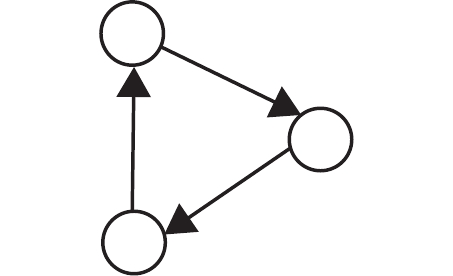$\sum\limits_{i,j,k}{{y}_{ij}}^{t}{{y}_{jk}}^{t}{{y}_{ki}}^{t}$ $\sum\limits_{i,j,k}{{y}_{ij}}^{+}\max ({{y}_{ki}}^{+},{{y}_{jk}}^{+})$ 传递闭合性（Transitivity） 网络中三个节点的循环关系，其中点i连接到j，点j连接到k，点i连接到k$\sum\limits_{i,j,k}{{y}_{ij}}^{t}{{y}_{jk}}^{t}{{y}_{ik}}^{t}$ $\sum\limits_{i,j,k}{{y}_{ij}}^{+}\max ({{y}_{ki}}^{+},{{y}_{kj}}^{+})$ 同配性（Homophily） 检验网络中属性相同的国家是否倾向于发生贸易关系$\sum\limits_{i,j}{{y}_{ij}}^{t}|{{\delta }_{i}}^{t}-{{\delta }_{j}}^{t}|$ $\sum\limits_{i,j}{{y}_{ij}}^{+}|{{\delta }_{i}}^{+}-{{\delta }_{j}}^{+}|$ 发送者效应（Sender） 检验网络中具有某种属性的国家是否对外建立出口关系$\sum\limits_{i,j}{{y}_{ij}}^{t}{{\delta }_{i}}^{t}$ $\sum\limits_{i,j}{{y}_{ij}}^{+}{{\delta }_{i}}^{t}$ 外生网络效应（Edgecov） 检验在其他网络中存在关系是否更容易产生贸易关系$\sum\limits_{i,j}{{y}_{ij}}^{t}{{x}_{ij}}^{t}$ $\sum\limits_{i,j}{{y}_{ij}}^{+}{{x}_{ij}}^{t}$ 稳定性（Stability） 检验t−1期的网络格局是否 影响t期的网络格局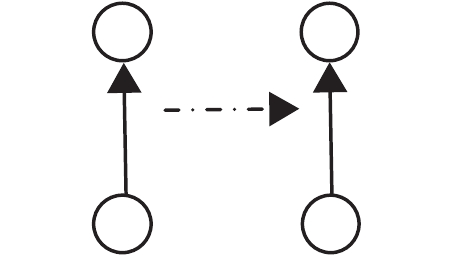$\sum\limits_{i\ne j}{{y}_{ij}}^{t}{{y}_{ij}}^{t-1}+(1-{{y}_{ij}}^{t})(1-{{y}_{ij}}^{t-1})$

2. 行为者-关系效应变量。网络中个体的一些品质或属性对于网络关系的形成非常重要。在贸易网络中，国家的要素禀赋、经济发展水平或其他特征对贸易关系的形成或维持也具有影响，这些特征通过“行为者属性”来测量，在指数随机图模型中也被称为“行为者—关系”效应，指特定国家属性对贸易关系产生的影响。本文中的行为者-关系效应包括同配性和发送者效应。其中，同配性（Homophily）用于描述具有相同属性的国家形成贸易关系的可能性，发送者效应（Sender）用来描述具有某种属性的国家与别国建立出口贸易关系的可能性。正的同配性效应反映了具有相同属性的国家之间容易形成或维持贸易关系，负的同配性效应则反映了具有相同属性的国家之间不容易形成或维持贸易关系；正的发送者效应反映了具有某种属性的国家容易对外发送出口贸易关系。根据贸易引力模型原理，两国的经济发展水平是影响两国贸易的重要因素，因此本文将经济发展水平作为国家属性。

3. 外部网络效应变量。除了内生结构效应和行为者-关系效应外，其他的外生情境因素对于网络的形成也具有一定的影响。贸易网络中的国家之间可能还存在其他类型的网络关系，而外部网络效应就决定了贸易网络关系与其他网络关系之间的共生程度。传统的引力模型认为，地理距离会对两国间的贸易产生负面影响，因此本文将各国间的地理距离构成的加权网络作为外生网络嵌入模型，以检验其影响程度。地理距离的负效应反映出两国距离越远，建立或维持贸易关系的概率就越小。图 1 “一带一路”沿线国家货物贸易网络关联图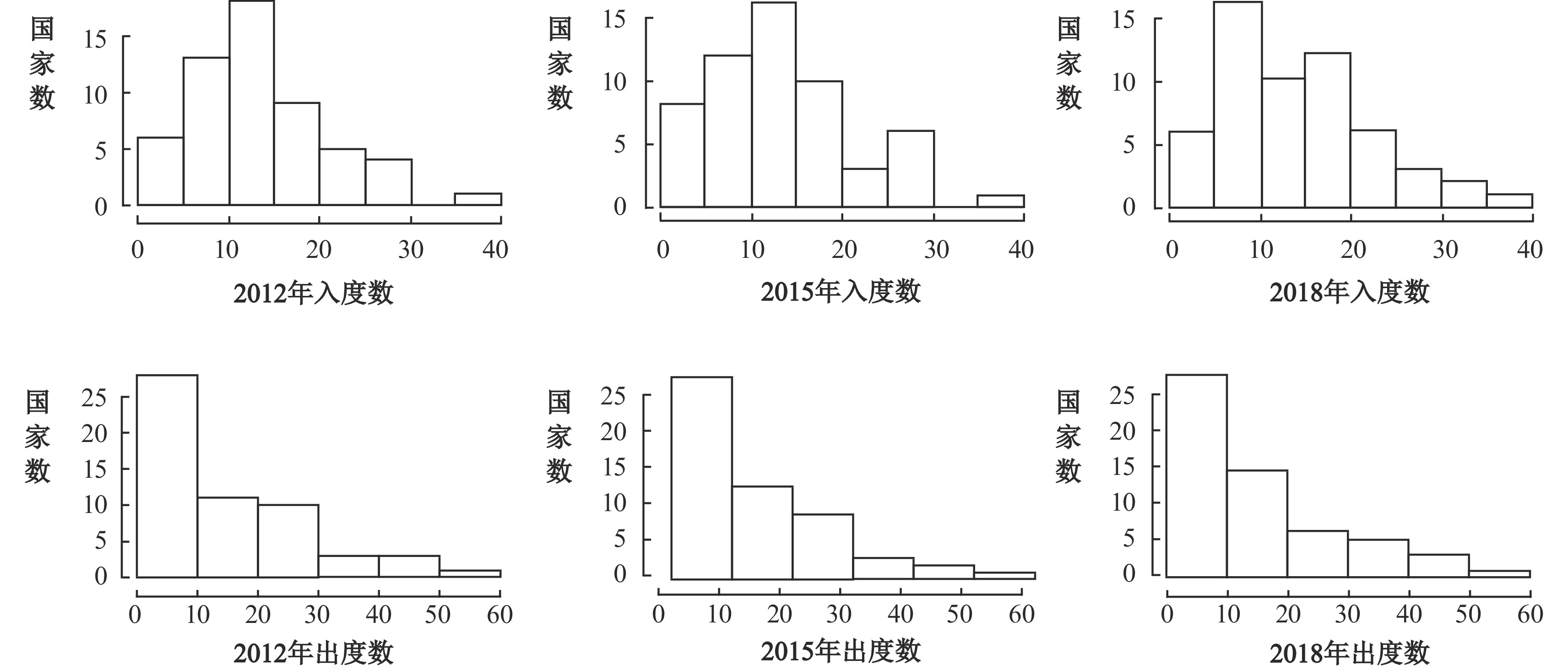图 2 “一带一路”沿线国家货物贸易网络入度与出度分布图

（一）数据来源及处理

（二）实证结果

 网络统计量 ERGM TERGM Edges −3.966***（0.362） −2.709***（0.525） （Delayed）Reciprocity 2.689***（0.104） 1.414***（0.132） Cyclicity −1.089***（0.053） −0.463***（0.060） Transitivity 2.736***（0.343） 1.997***（0.515） Homophily（High GDP） 0.800***（0.070） 0.434***（0.147） Homophily（Low GDP） −0.911***（0.115） −0.723***（0.219） Sender（High GDP） 0.401***（0.082） 0.367***（0.153） Stability 2.218***（0.058） 注：括号内为标准差；***、**和*分别表示在1%、5%和10%水平上显著。下同。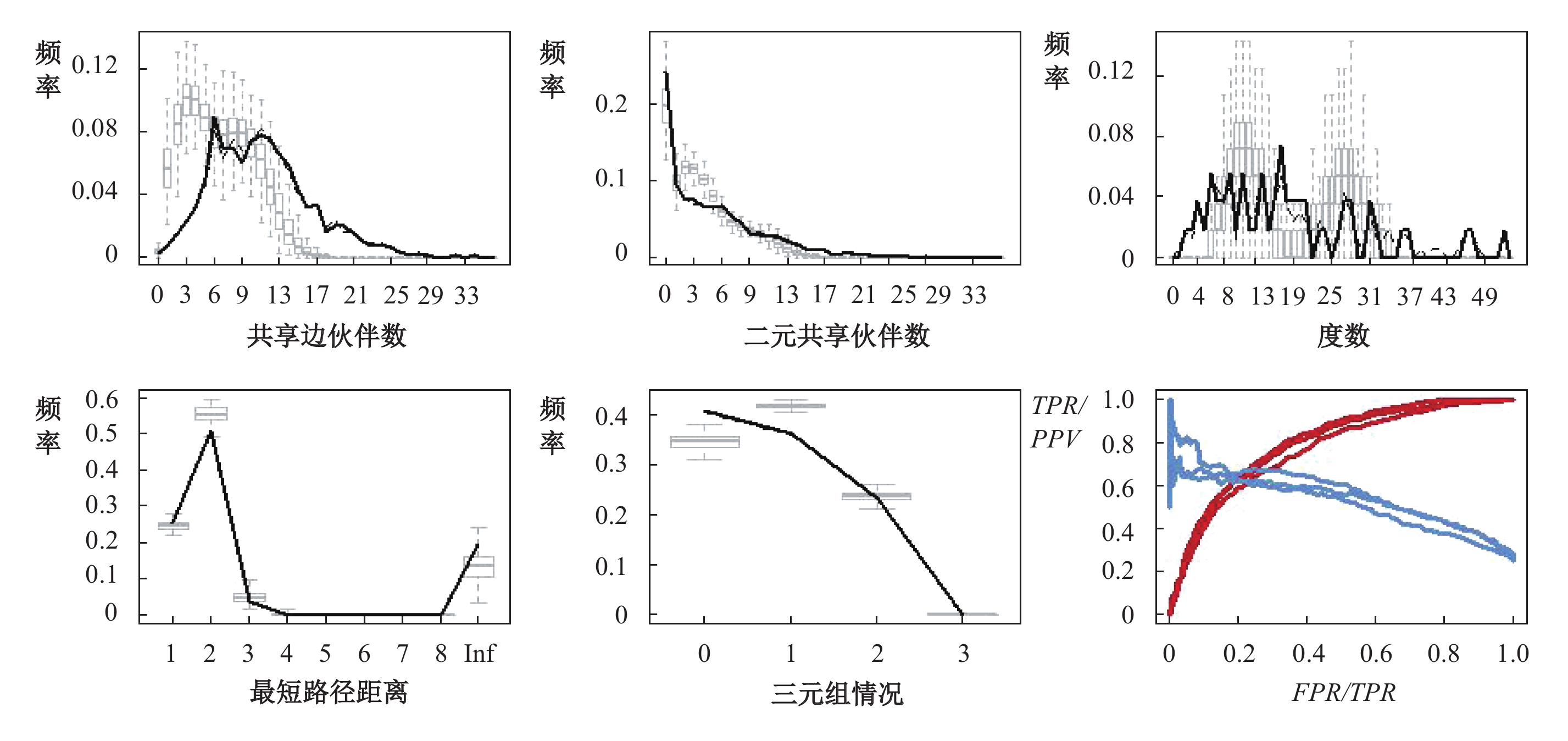图 3 ERGM的拟合效果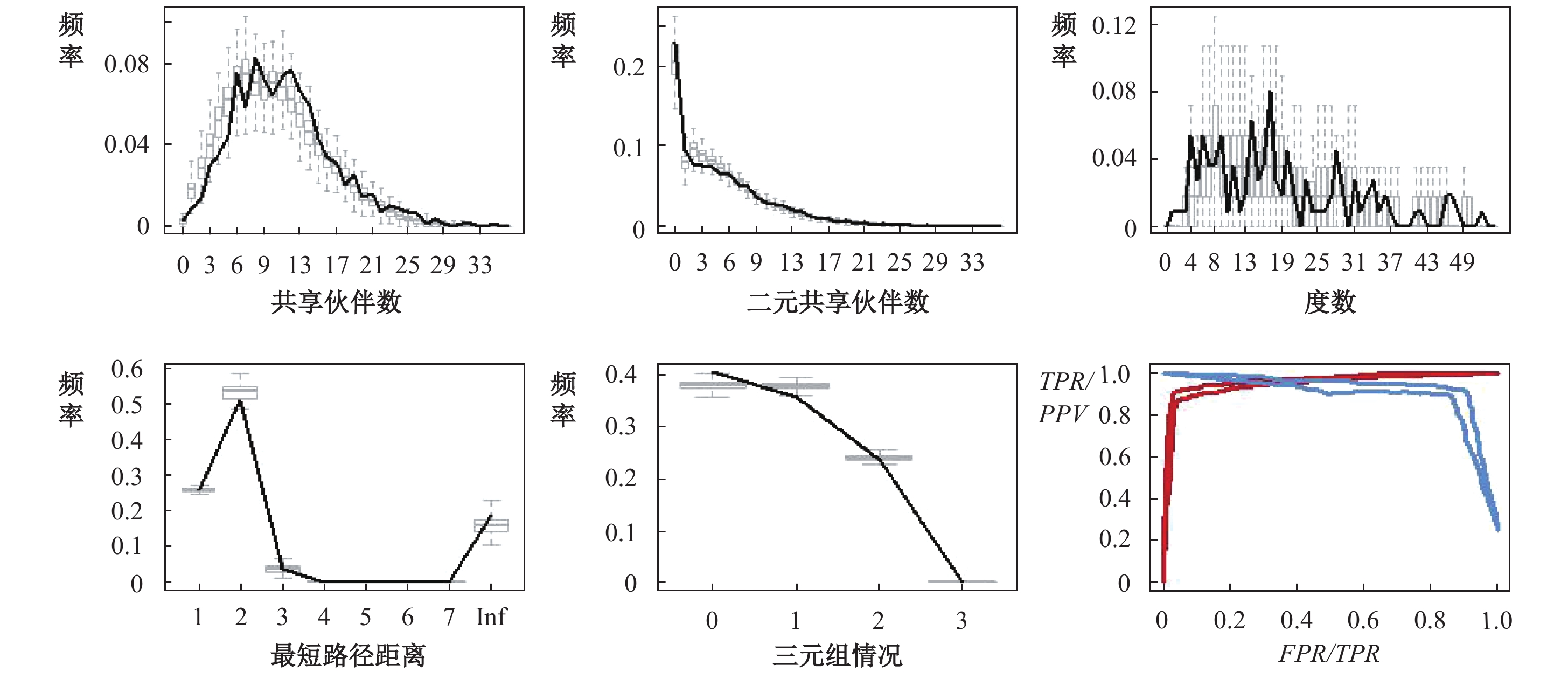图 4 TERGM的拟合效果

 网络统计量 TERGM STERGM Formation Dissolution Edges −2.709***（0.525） −4.275***（0.462） 0.573 （0.638） （Delayed）Reciprocity 1.414***（0.132） 1.649***（0.183） 1.476***（0.214） Cyclicity −0.463***（0.060） −0.346***（0.056） −1.020***（0.113） Transitivity 1.997***（0.515） 1.129**（0.440） 1.445**（0.639） Homophily（High GDP） 0.434***（0.147） 0.603***（0.203） 0.184 （0.225） Homophily（Low GDP） −0.723***（0.219） −1.587***（0.369） −0.034 （0.352） Sender（High GDP） 0.367***（0.153） 0.224 （0.201） 0.510*（0.262） Stability 2.218***（0.058）

 网络统计量 TERGM STERGM Formation Dissolution Edges −2.310***（0.069） −3.856***（0.061） 1.498***（0.064） （Delayed）Reciprocity 1.427***（0.024） 1.757***（0.044） 1.050***（0.036） Cyclicity −0.424***（0.074） −0.424***（0.077） −0.266***（0.067） Transitivity 1.829***（0.070） 0.825***（0.068） 0.458***（0.067） Homophily（High GDP） 1.234***（0.028） 1.407***（0.065） 1.335***（0.007） Homophily（Low GDP） −0.959***（0.016） −1.740***（0.010） −0.599***（0.019） Sender（High GDP） 0.380***（0.069） −0.132 （0.080） 0.748***（0.025） Edgecov（Distance net） −1.653***（0.385） −0.989**（0.049） −2.370***（0.552） Stability 2.429***（0.087）

 网络统计量 TERGM STERGM Formation Dissolution Edges −2.131***（0.047） −4.097***（0.051） 0.167**（0.071） （Delayed）Reciprocity 1.334***（0.020） 1.475***（0.026） 1.547***（0.026） Cyclicity −0.548***（0.076） −0.301***（0.075） −1.495***（0.085） Transitivity 1.978***（0.047） 1.443***（0.054） 2.610***（0.069） Homophily（High GDP） 0.087***（0.032） 0.225***（0.043） −0.161*（0.083） Homophily（Low GDP） −0.853***（0.010） −1.575***（0.008） 0.755***（0.043） Sender（High GDP） 0.316***（0.074） 0.434***（0.081） 0.576***（0.110） Edgecov（Distance net） −0.626**（0.312） −0.686***（0.350） −0.656 （0.451） Stability 2.029***（0.074）

（三）TERGMSTERGM的稳健性检验

① 参见《推动共建丝绸之路经济带和21世纪海上丝绸之路的愿景与行动》，2015年3月28日。

② 参见《“一带一路”贸易合作大数据报告2018》，2018年5月6日。

③ 描述网络特征的指标主要包括节点度分布、中心性、互惠性、聚集性等基本指标。节点度分布描述了网络中节点接受或发送关系的情况；节点中心性衡量了网络中节点在影响其他点之间关系时发挥的作用；互惠性和聚集性指标分别衡量了网络全局的互惠性和聚集性，即网络中关系在多大程度上是闭合的和互惠的。

④ 数据来源于中华人民共和国商务部网站（http://www.mofcom.gov.cn/）和中国一带一路网（https: //www.yidaiyilu.gov.cn/）。

⑤ 参见[澳]迪安·鲁谢尔、[英]约翰·科斯基宁、[澳]加里·罗宾斯：社会网络指数随机图模型：理论、方法与应用，社会科学文献出版社2016年版，第11页。

⑥ 参见Krivitsky P N，Handcock M S：A Separable model for dynamic networks，Journal of the Royal Statistical Society Series B（Statistical Methodology），2014。

STERGM中关系生成模型（Formation）采用含y+的动态变量，关系消失模型（Dissolution）采用含y-的动态变量，表中以Formation模型为例，Dissolution模型同理。

⑧ 本文也尝试着对“一带一路”三条推进路径构成的分网络进行实证检验，但由于分样本情况下样本量较少，部分网络过于稀疏，网络结构特征不明显，且模型待估参数较多，导致参数估计不收敛，因此本文没有考虑“一带一路”三条推进路径下的情况。

ERGM对多期数据的拟合实际上是在多个截面上对数据分别进行拟合并求其平均效应，而STERGM由于本质上是将TERGM拆分为两部分，不易对比结果，因此在对比时没有加入STERGM方法。

  [澳]迪安•鲁谢尔, [英]约翰•科斯基宁, [澳]加里•罗宾斯. 社会网络指数随机图模型: 理论、方法与应用[M]. 杜海峰, 任义科, 杜巍, 等译. 北京: 社会科学文献出版社, 2016.  陈银飞. 2000−2009年世界贸易格局的社会网络分析[J]. 国际贸易问题, 2011(11): 31–42.  李敬, 陈旎, 万广华, 等. “一带一路”沿线国家货物贸易的竞争互补关系及动态变化——基于网络分析方法[J]. 管理世界, 2017(4): 10–19.  许和连, 孙天阳, 成丽红. “一带一路”高端制造业贸易格局及影响因素研究——基于复杂网络的指数随机图分析[J]. 财贸经济, 2015(12): 74–88.  郑军, 张永庆, 黄霞. 2000-2014年海上丝绸之路贸易网络结构特征演化[J]. 国际贸易问题, 2017(3): 154–165.  邹嘉龄, 刘卫东. 2001～2013年中国与“一带一路”沿线国家贸易网络分析[J]. 地理科学, 2016(11): 1629–1636.  Cranmer S J, Heinrich T, Desmarais B A. Reciprocity and the structural determinants of the international sanctions network[J]. Social Networks, 2014, 36: 5–22. DOI:10.1016/j.socnet.2013.01.001  Desmarais B A, Cranmer S J. Statistical mechanics of networks: Estimation and uncertainty[J]. Physica A: Statistical Mechanics and its Applications, 2012, 391(4): 1865–1876. DOI:10.1016/j.physa.2011.10.018  Fritz C, Lebacher M, Kauermann G. Tempus volat, hora fugit: A survey of tie-oriented dynamic network models in discrete and continuous time[J]. Statistica Neerlandica, 2019(3): 1–47.  Hanneke S, Fu W J, Xing E P. Discrete temporal models of social networks[J]. Electronic Journal of Statistics, 2010, 4: 585–605. DOI:10.1214/09-EJS548  Ingold K, Leifeld P. Structural and Institutional Determinants of influence reputation: A comparison of collaborative and adversarial policy networks in decision making and Implementation[J]. Journal of Public Administration Research and Theory, 2016, 26(1): 1–18. DOI:10.1093/jopart/muv041  Krivitsky P N, Handcock M S. A separable model for dynamic networks[J]. Journal of the Royal Statistical Society: Series B（Statistical Methodology）, 2014, 76(1): 29–46. DOI:10.1111/rssb.12014  Leifeld P, Cranmer S J, Desmarais B A. Temporal exponential random graph models with btergm: Estimation and bootstrap confidence intervals[J]. Journal of Statistical Software, 2018, 83(6): 1–36.